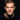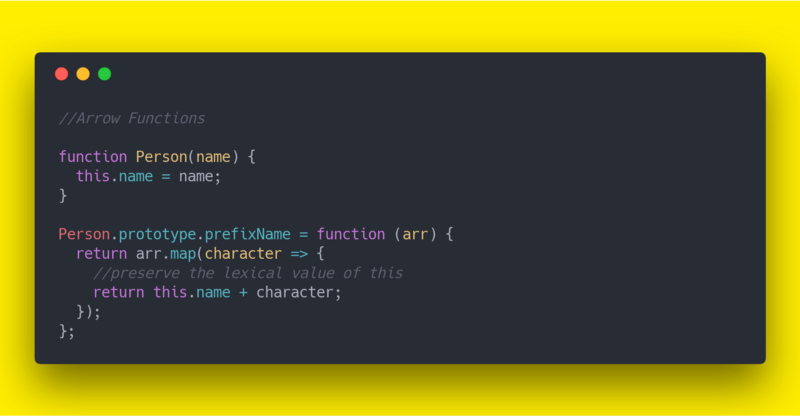# ES6 cheatsheet  —  Arrow FunctionsSerban Mihai / 20 October 2018Arrows are a function shorthand using the `=>` syntax. Arrow functions allow you to preserve the lexical value of `this`.

Take the example below where we have a nested function, in which we would like to preserve the context of `this` from its lexical scope:

``````function Person(name) {
this.name = name;
}

Person.prototype.prefixName = function (arr) {
return arr.map(function (character) {
return this.name + character; // Cannot read property 'name' of undefined
});
};``````

Using Arrow Functions, the lexical value of `this` isn't shadowed and we can re-write the above as shown:

``````function Person(name) {
this.name = name;
}

Person.prototype.prefixName = function (arr) {
return arr.map(character => this.name + character);
};``````

If an arrow is inside another function, it shares the `arguments` variable of its parent function. Example:

``````// Lexical arguments
function square() {
let example = () => {
let numbers = [];
for (let number of arguments) {
numbers.push(number * number);
}

return numbers;
};

return example();
}

square(2, 4, 7.5, 8, 11.5, 21); // returns: [4, 16, 56.25, 64, 132.25, 441]``````

You can find a more complete ES6 cheetsheet on my Github page.++= ❤️[Source code]